For the level shift, it has been implicitly presumed that vibronic transitions always take place at equilibrium configurations of the defects. Thereby, it has been ruled out that the defects are thermally excited2 up to the intersection points of their adiabatic potentials (see Fig. 2.4). However, this case has been accounted for in the nonradiative multi-phonon theory (NMP) . This mechanism has already been suggested for random telegraph noise and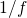noise in microelectronic devices , where only a simplified description of NMP process has been employed. Furthermore, it is also encountered in the context of phonon-assisted tunneling ionization of deep centers  and discussed on various levels of theoretical sophistication  including additional second-order effects, such as the Coulomb energy  and field-enhancement factors .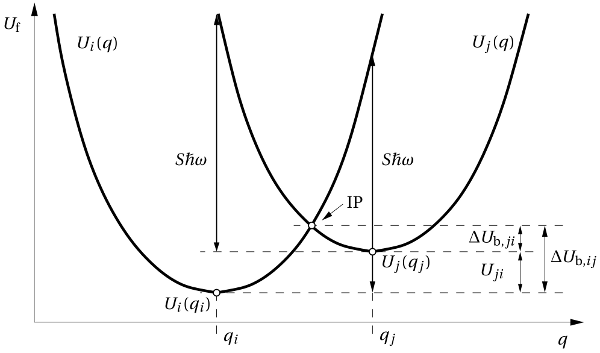Figure 2.4: The configuration coordinate diagram for the NMP model. The two parabolas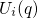and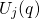show the adiabatic potentials for the case that the electron is located in the channel or the defect, respectively. IP labels the intersection point ofand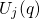, where the NMP process takes place in the classical approximation. The electron capture or emission rates are primarily determined by the NMP barriers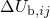and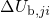, respectively.

In NMP theory, the equation (2.21) is generalized to account for all possible thermal excitations. Then the equation (2.21) must be rewritten as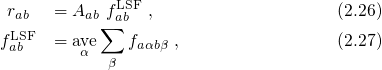where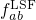is referred to as the lineshape function. ‘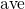’ denotes the thermal average over all initial vibrational states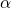and accounts for the thermal excitations using a sum over weighted Boltzmann factors. The lineshape function eventually depends on the Franck-Condon factor and thus on the complicated shape of the adiabatic potentials of the defects. It is noted that these potentials are not assessable via experiments but can also not be calculated using first-principles calculations (see Section 3.3), which would by far exceed the current computational capabilities. However, they can be reasonably approximated using the harmonic approximation when only small displacements from the equilibrium configuration of the defects are considered. In this approximation, the adiabatic potentials are represented as a Taylor expansion whose linear term vanishes close to the equilibrium configuration. As a result, these potentials become parabolic and therefore, describe harmonic oscillators frequently used in solid state physics. A corresponding configuration coordinate diagram for the vibronic transitions of a defect is depicted in Fig. 2.4. The total energies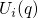and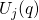in Fig. 2.4 include the contributions from the defect atoms along with its immediate surrounding (and the channel region) and therefore correspond to the adiabatic potentials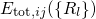. For this reason, their corresponding adiabatic potentials differ only in the location of electron involved in the trapping process. In the case of, the electron resides in the channel while, for, it is located at the defect site. In the harmonic approximation, the adiabatic potentials can be written as: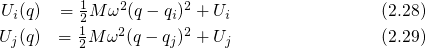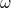stands for the vibrational frequency of the oscillator and determines the curvature of the parabola while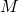is the mass of the oscillator. Analogously to the previous section, the classical vibronic transitions are assumed to occur at the intersection points of the adiabatic potentials. Therefore, the defect system must be thermally excited from its initial configuration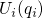to the intersection point IP of the two parabolas in Fig. 2.4. At this point, the total energiesand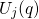equal and allow for an elastic tunneling transition. From there, the system relaxes to the equilibrium configuration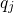with the energy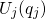. The energy difference betweenandcan be expressed as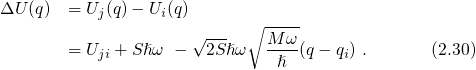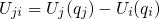is the so-called thermodynamic trap level. It is given relative to the conduction or the valence band edge for electron or hole trapping3 and determines the occupancy of the defect in thermal equilibrium. As demonstrated in Fig. 2.4, the Huang-Rhys factor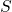is defined by the energy differences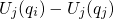and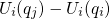, which are both equivalent tophonons with an energy of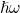. That is, this quantity determines the intersection point of the parabolas and eventually impacts the probability for an electron transition between the defect and the channel. In the above NMP concept, it has been assumed that the charge state of the defect does not affect the curvatures ofand. As a result, only a linear term in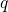appears in equation (2.30). Note that its sign is determined by the relative positions of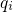andbut has no physical meaning. The forward and reverse barrier of this process are given by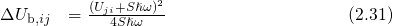and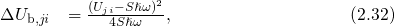respectively.

The NMP mechanism was suggested several decades ago but has been disregarded in the context of NBTI so far. Nevertheless, this mechanism should be considered as a possible description of charge trapping in NBTI. The underlying theory relies on the complicated quantization effects of the nuclei system and is therefore quite complex in its original variant. However, several convenient and accurate approximations, including the version presented in this section, have been developed over the years and allow for theoretical investigations on a device level.